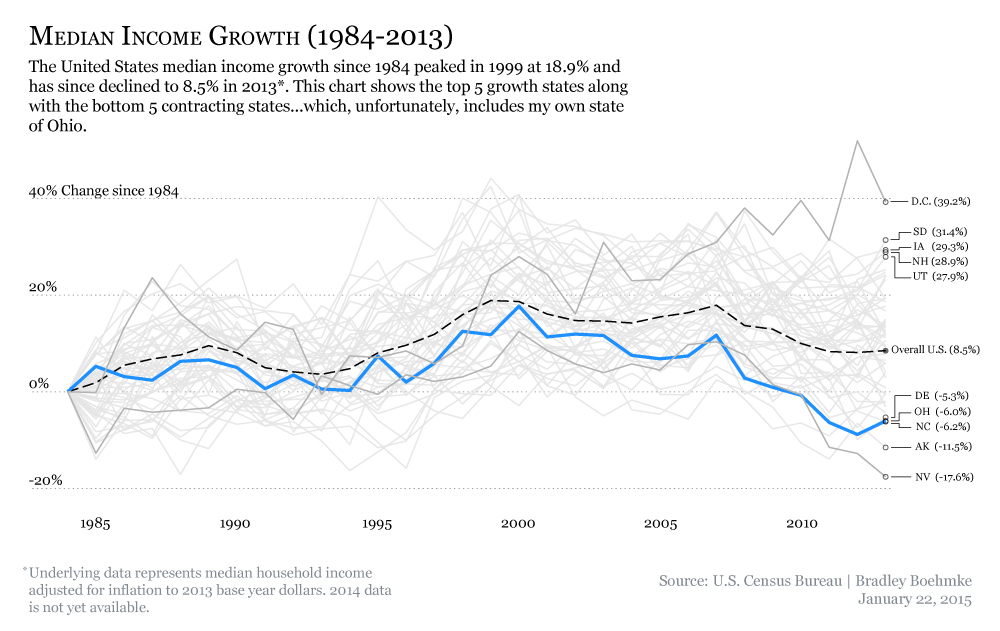Disclaimer: I, by no means, consider myself a programmer but, rather, a novice to coding…errrr…hacking to solve my problems. I do not claim that the code presented below is the “best” code nor the most efficient so I welcome comments and suggestions for improvements but discourage unpleasantries

This report provides the code behind the visual graphic created to illustrate the historical growth rates of median income in the United States.#### R Packages Utilized

``````# Preprocessing & summarizing data
library(dplyr)
library(tidyr)

# Visualizatin development
library(grid)
library(scales)
library(ggplot2)``````

#### Getting & Preparing the Data

The data used was obtained from the U.S. Census Bureau website which contains median income for all 50 U.S. states, D.C., and the overall U.S. median income. The values have been inflation adjusted to 2013 constant year dollars and range from 1984-2013. The raw data included standard errors for each year which I removed prior to reading into R. So, the initial data I am starting with here looks like:

``````##           State X2013 X2012 X2011 X2010 X2009 X2008 X2007 X2006 X2005
## 1 United States 51939 51758 51842 52646 54059 54423 56436 55689 55278
## 2       Alabama 41381 44096 44112 43733 43420 48119 47424 43848 44329
## 3        Alaska 61137 64573 59483 61804 66904 69230 70771 65183 66691
## 4       Arizona 50602 47728 50358 50103 49674 50757 53045 53905 53988
## 5      Arkansas 39919 39585 42778 41226 39681 42828 45832 42814 43742
## 6    California 57528 57849 55274 57996 60963 61684 62616 63913 61756``````

The first step I took was to create my baseline comparison which is the overall U.S. median income. This simply required me to filter out values only for the U.S. (labeled “United States”), transpose the data into a long format using `gather()`, and removing the unecessary “X” at the beginning of each year value by using `separate()`.

``````# clean overall U.S. data and turn it into long format
us <- data %>%
filter(State == "United States") %>%
gather(Year, Income, X2013:X1984) %>%
separate(Year, c("left","Year"), sep="X") %>%
select(-left) %>%
arrange(Year)

# extract 1984 value as the baseline value and add to us dataframe
us_base <- us[us\$Year==1984,3]
us\$us_baseline <- us_base

# calculate the percent change in U.S. median income for each year as compared
# to 1984 (aka the baseline)
us <- us %>% mutate(us_change = (Income-us_baseline)/us_baseline)``````

The second step is to do the same process but for each of the states.

``````# create a states dataframe, clean and turn into long format
states <- data %>%
filter(State != "United States") %>%
gather(Year, Income, X2013:X1984) %>%
separate(Year, c("left","Year"), sep="X") %>%
select(-left) %>%
arrange(Year) %>%
filter(Income != "NA")

# create baselines for each state
state_base <- states %>%
filter(Year == 1984) %>%
select(State, State_Baseline = Income)

# add baseline to the states and calculate the percent change in median income
# for each state as compared to 1984 (aka the baseline)
states <- states %>%
left_join(state_base) %>%
arrange(State) %>%
mutate(state_change = (Income-State_Baseline)/State_Baseline)

# change year variables from character to numeric
states\$Year <- as.numeric(states\$Year)
us\$Year <- as.numeric(us\$Year)

# get top 5 and bottom 5 states which will allow me to identify them
# graphically
rank <- states %>%
filter(Year == 2013) %>%
arrange(desc(state_change)) %>%
mutate(rank = seq(1,length(State), by=1)) %>%
filter(rank < 6 | rank > 46 )``````

#### Creating the Visual Graphic

Now that we have our data formatted properly we can proceed to building our graphic.

##### Step 1

The first thing I like to do is create my canvas. I tend to stay true to Edward Tufte’s principles and keep it as minimalistic as possible. This usually includes the `theme_bw()` theme along with minimal gridlines. For this plot I also remove the borders, axis tick marks, titles, and make the y-axis major gridlines a light grey with a dotted linetype. Next, I plot the trend lines for all 50 states (and D.C.). Since this creates a lot of noise, I use a light grey with some transparency because I want this to act as a “backdrop” of sorts.

``````p <- ggplot(states, aes(Year, state_change, group=State)) +
theme_bw() +
theme(plot.background = element_blank(),
panel.grid.minor = element_blank(),
panel.grid.major.x = element_blank(),
panel.grid.major.y = element_line(linetype = 3, colour = "grey50"),
panel.border = element_blank(),
panel.background = element_blank(),
axis.ticks = element_blank(),
axis.title = element_blank()) +
geom_line(colour="grey90", alpha=.9)

print(p)``````